# Lewis Acid and Base Lab Report

4333 words (17 pages) Essay

18th May 2020 Chemistry Reference this

Disclaimer: This work has been submitted by a university student. This is not an example of the work produced by our Essay Writing Service. You can view samples of our professional work here.

Any opinions, findings, conclusions or recommendations expressed in this material are those of the authors and do not necessarily reflect the views of UKEssays.com.

Introduction

A majority of chemical reactions involve electrons interacting between compounds. It is important to picture these interactions through molecular orbital (MO) theory, where for Lewis acids and bases the movement of electrons between HOMOs (Highest occupied molecular orbital) and LUMOs (Lowest unoccupied molecular orbital). In the real world, some examples of acid and base reactions are baking soda applications for cooking and acid indigestion, and kitchen cleaning supplies. The energy gap between HOMOs and LUMOs is often low, allowing the movement of electrons from HOMO to LUMO to proceed with ease.

If you need assistance with writing your essay, our professional essay writing service is here to help!

Borane (BH3), as a Lewis acid does not satisfy the octet rule and has electron deficiency. It also shows an odd case of existing in equilibrium with B2H6, a Lewis acid-base adduct between two boranes.Borohydride (BH4) can act as a Bronsted-Lowry base (proton acceptor) with acids (proton donor). However, unlike borohydride, BH3 and B2H6 is a Lewis acid (electron pair donor) and it donates electron lone pairs to empty orbitals of bases (electron pair acceptors).

IR spectroscopy is a powerful tool used to identify bond stretches for analytes. It also has practical uses for isotope labeling experiments. Several factors relating to equations 1-5 are taken into account for IR stretching. These equations are further explained and applied in the results and discussion section of the paper.One aspect of IR stretching relates to Hooke’s law, where it explains the force for the stretch directly proportional to amount of stretch. Since the amount of stretch depends on the mass of atom which decides the bending and stretching of bond, Hooke’s law (1) is applied to measure potential energy E.

Figure 1 mechanistically shows how BH4 reacts with tBuNH3Cl to form H3B-NH2tBu. BH4 acts as a Bronsted Lowry base, where the hydrogen atom accepts a proton to form hydrogen gas.

E(x) = $\frac{{1}}{{2}}{k}{{x}}^{{2}}$

(k = force constant) (1)

${{E}}_{{n}}\left({n}{+}\frac{{1}}{{2}}\right){\mathit{hv}}{=}\left({n}{+}\frac{{1}}{{2}}\right){\mathit{hv}}{=}\left({n}{+}\frac{{1}}{{2}}\right){h}\left(\frac{{1}}{{2}{\pi }}\right)\sqrt{\frac{{k}}{{u}}}{=}\left({n}{+}\frac{{1}}{{2}}\right){\mathit{hw}}$

(2)

(n = 0,1,2,….)

ω = vibrational frequency ( $\sqrt{\frac{{k}}{\mathrm{µ}}}{\right)}$

(3)

$\mathrm{µ}$

(reduced mass) = $\frac{{{m}}_{{A}}{*}{{m}}_{{B}}}{{{m}}_{{A}}{+}{{m}}_{{B}}}$

(4)

$\frac{{v}{\left(}{\mathit{BD}}{\right)}}{{v}{\left(}{\mathit{BH}}{\right)}}{=}\sqrt{\frac{{u}{\left(}{\mathit{BH}}{\right)}}{{u}{\left(}{\mathit{BD}}{\right)}}}$

(5)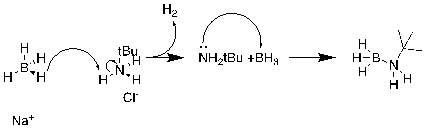Figure 1) Mechanism of reaction of BH4 and tBuNH3Cl

Experimental

All chemicals and instruments were provided from the IU chemistry department and chemicals were used without any further purification. Spectrum 100 FT-IR spectrometer from Perkin Elmer was used for IR spectroscopy. Spartan 16 was used for computational analysis.

Synthesis of the Lewis Acid-Base Adduct

Tert-butyl ammonium chloride (1.304 g, 0.012 mol) was added to THF (15 mL) were stirred at room temperature. Powdered NaBH4 (0.200 g, 0.0053 mol) was added during mixing, where gas evolution (H2) was observed. While mixing, Additional THF (10 mL) was added to the mixture and was stirred for an additional 1.5 hours and filtered with suction filtration for removal of insoluble materials. 5 drops of the solution were added to a salt plate (KBr) for IR spectroscopy. IR (KBr, cm-1): 2656, 2554, 2452, 1837, 1552, 1349, 1227, 1065, 460. The melting point of Duetero was 53.2-102 ${℃}$

and Melting point of Proteo was 82-96 ${℃}$

for the product. For isotope labeling analysis, NaBD4 (0.210 g, 0.0056 mol) was added in place of Na BH4 and followed identical procedures, where gas evolution (DH) was also observed.

Result and discussion

 Compound Orbital Energy (eV) Figure BH3 LUMO -1.8eV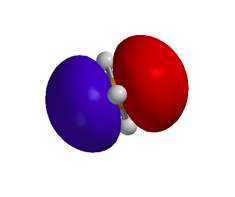BF3 LUMO 1.0eV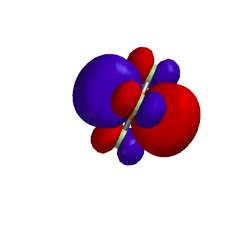tBuNH2 HOMO -6.2eV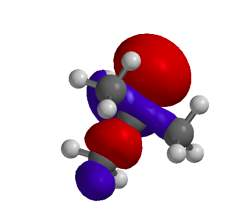N(CH3)3 HOMO -5.6eV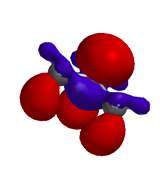Table 1) Energy level calculations HOMO and LUMO of BH3, BF3, tBuNH2, and N(CH3)3 Data were analyzed based on the Spartan calculations.

Borane (BH3) and boron triflouride (BF3) were defined as Lewis acids considering their ability to accept electrons. Both BH3 and BF3 have vacant electron sites, and they accept electrons from the HOMO of Lewis bases to fill their empty pi orbitals. On the contrary, tBuNH2 and N(CH3)3 were identified as Lewis bases due to their rich electron environments.

The LUMO energy of BH3 (-1.8 eV) was lower than BF3 (1.0 eV). Because BF3 is stronger acid than BH3. Fluorine is more electronegative than hydrogen, causing inductive effects on boron, and the electron density of boron is lower in boron trifluoride compared to borane. Thus, the energy of BH3 is shown to be lower than BF3, and BF3 is considered to have greater acidity. The boron atom of BF3 is a better electron acceptor than for BH3.

The HOMO energy of tBuNH2 (-6.2 eV) was lower than that of N(CH3)3 (-5.6 eV). This difference in energy is explained by the lone pair of N(CH3)3 concentrated on nitrogen atom, while for tBuNH2, the electron density is distributed to the C-H bonds. Thus, N(CH3)3 has higher HOMO energies and its ability to donate electrons is greater.

tBuNH2, N(CH3)3 are Lewis bases with high HOMO energies, and donate electrons to borane and boron trifluoride to fill vacant electron sites. Lewis acids tend to accept electrons from Lewis bases to fill out empty sites of electrons and stabilizes the HOMO. LUMO has empty electron sites, so lower energy LUMOs indicate that Lewis acids are electrophilic, while higher energies of HOMOs indicate a compound being a Lewis base due to their active donation of electrons to the LUMO. Regarding this theory, bond interaction between the HOMO of tBuNH2 and the LUMO of BH3 is shown in Figure 2 in the form of an MO diagram.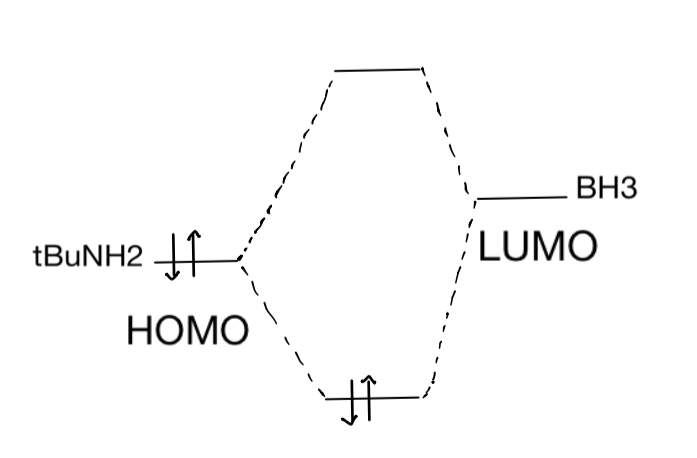Figure 2) tBuNH2 and BH3 MO diagram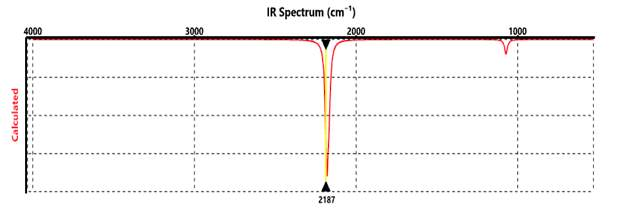Figure 3) Spartan IR spectrum (BH4): B-H stretch frequency 2187cm-1 (Highest Intensity)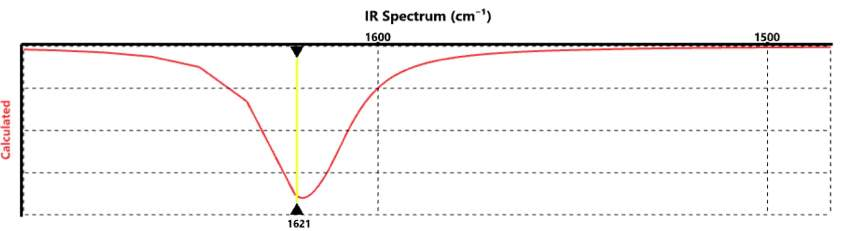Figure 4) Spartan IR spectrum (BD4): B-D Stretch Frequency: 1621 cm-1 (Highest Intensity)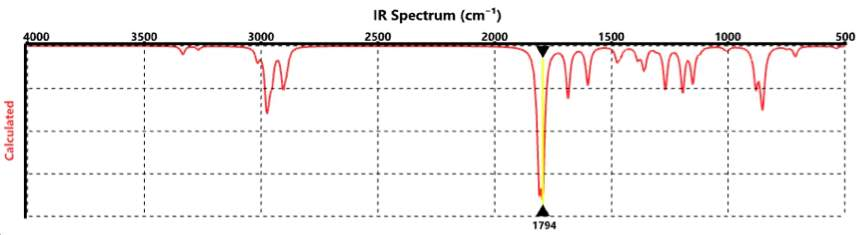Figure 5) Spartan IR spectrum (D3B-NH2(tBu)): B-D Stretch Peak: 1794 cm-1 (Highest Intensity)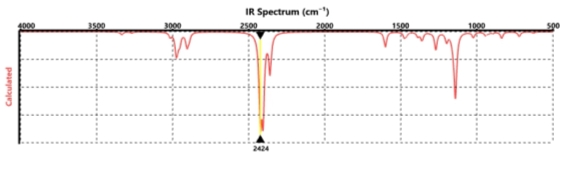Figure 6) Spartan IR spectrum (H3B-NH2(tBu)): B-H Stretch Frequency: 2424 cm-1 (Highest Intensity)

Computational isotopic labeling experiments were conducted and analyzed using Spartan IR calculations. For BD4, and BH4, the highest peak for the B-D stretch was 1621 cm-1 and for the B-H stretch was 2187 cm-1. Similar differences are shown for D3B-NH2(tBu) and H3B-NH2(tBu) as well. IR stretches for B-N around 1100 cm-1 to 800 cm-1 are observed for H3B-NH2(tBu) and D3B-NH2(tBu) as well. The difference between B-H and B-D peaks are explained using equations (4) and (5). Equation (4) shows the reduced mass calculation for boron and hydrogen. Equation (5) is used to compare when a particular peak of the IR spectrum arises predominantly from band stretching of B-H and B-D. Peaks corresponding to B-H and B-D showed different IR stretches and agreed with calculated estimations. Sample calculations of equations (4) and (5) are shown below.

${\mathrm{µ}}{=}\frac{{{m}}_{{B}}{*}{{m}}_{{H}}}{{{m}}_{{B}}{+}{{m}}_{{H}}}{=}\frac{{\left(}{10}{.}{81}{\right)}{\left(}{1}{.}{01}{\right)}}{\left({10}{.}{81}\right){+}{\left(}{1}{.}{01}{\right)}}{=}{0}{.}{92}$

(6)

$\frac{{v}{\left(}{\mathit{BD}}{\right)}}{{v}{\left(}{\mathit{BH}}{\right)}}{=}\sqrt{\frac{{u}{\left(}{\mathit{BH}}{\right)}}{{u}{\left(}{\mathit{BD}}{\right)}}}{=}\sqrt{\frac{{0}{.}{92}}{{1}{.}{74}}}{=}{0}{.}{727}$

(7)

(8)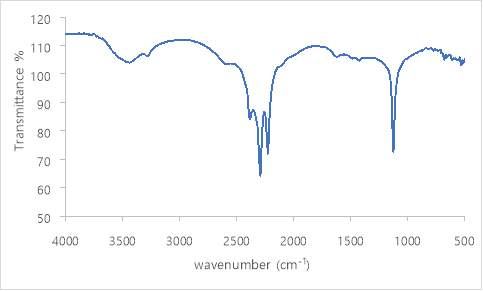Figure 6) IR spectrum of reactant B-H peak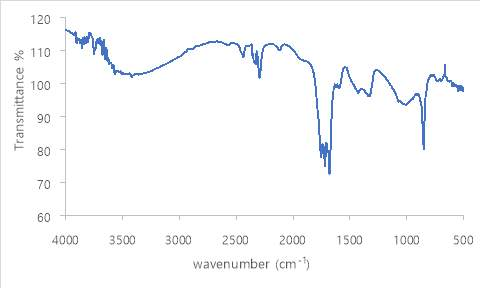Figure 7) IR spectrum of reactant B-D peak

Figure 8) IR spectrum of tBuNH2-BH3 peak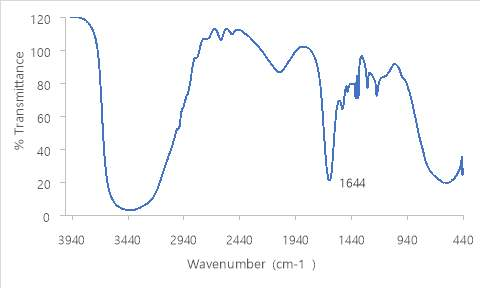Figure 9) IR spectrum of tBuNH2-BD3 peak

 Bond Stretch Wavenumber (cm-1) Experimental B-H (H3B-NH2tBu) 2274 B-D (D3B-NH2tBu) 1644 Actual B-H (H3B-NH2tBu) 2359 B-D (D3B-NH2tBu) 1634 Computational (Spartan) B-H (BH4) 2187 B-D (BD4) 1621 B-H (H3B-NH2tBu) 2424 B-D (D3B-NH2tBu) 1794

Table 2) Bond stretch wavenumber values

The ratio from the actual B-H stretch (2359 cm-1) to experimental B-H stretch (2410 cm-1) was 0.98, which was reasonable considering actual to experimental ratio values under 1.7 to be acceptable. The ratio of the actual B-D peak (1634 cm-1) to the experimental B-D peak (1644 cm-1) was also reasonable at 0.99. These ratio values indicate reliable experiment results, while minor shifts of IR peaks have been observed due to hydrated samples.

Our academic experts are ready and waiting to assist with any writing project you may have. From simple essay plans, through to full dissertations, you can guarantee we have a service perfectly matched to your needs.

The shift in wavenumbers correlate to changes in molecular interactions. Stronger bonds and lighter molecules will absorb at higher frequencies, while heavier molecules absorb at lower frequencies. Thus, B-H bonds will absorb at higher frequencies and B-D bonds absorb at lower frequencies. Equation (5) shows how differences in mass cause these shifts and allows successful estimations of how much the ratio is.

Comparing the data with groups’ NaBD4 IR spectrum data, it was found that the other group’s B-D peaks were sharper and had minimal to no THF and water peaks shown in the IR spectrum. The cause of such error was created due to excessive hydration of THF on the KBr plate. To effectively filter impurities, excessive time lengths of suction filtration were performed in addition to use of excessive amounts of THF. A large region around 3440 cm-1 corresponding to THF was observed along with huge water peaks inevitably due to wet KBr as observed in the blank sample.

 $v$ Ratios Experimental 0.72 Computational (Spartan) 0.741

Table 3) $v$

Ratios for B-D to B-H stretches (Calculations follow equation (8))

Ratios for the IR stretches obtained experimentally agree with the theoretical values. However, differences in ratios for the obtained experimental data were due to impurities and unreacted reagents. More sophisticated separation methods such as column chromatography need to be employed to successfully purify samples. These calculations also assume ideal gas phase reactions and fails to account for interactions with the solvent.

 Experimental Actual Initial temperature ( ${℃}{\right)}$ Final temperature ( ${℃}{\right)}$ D3B-NH2tBu 53.2 102 98 ${℃}$

Table 4) Melting point of D3B-NH2tBu

The melting point was not accurate enough due to impurity and fast evaporation. The range between the initial and final temperature was significantly large, indicating impure samples. However, the range falls within the actual melting point of the product.

Conclusion

A series of experiments have been performed to understand reactions of Lewis acid and base by relating to the HOMOs and LUMOs of certain chemicals. By reacting a Lewis acid and base through chemical synthesis and analyzing the reaction by means of IR spectroscopy and computational studies, an understanding of electron exchange between chemicals is solidified. IR and computational data support proof of how Lewis acids such as BH3 tend to accept electrons and bases like tBuNH2 may act as electron donors.

References

 Calbreath, D. Lewis acids and bases. cK12

 Nave, R. Elastic potential Energy. HyperPhysics

 Andy, S. Principles of General Chemistry. 2012

 Ryu, R. S. Noda I. Jung, Y. M. American Laboratory. 2011

Introduction

A majority of chemical reactions involve electrons interacting between compounds. It is important to picture these interactions through molecular orbital (MO) theory, where for Lewis acids and bases the movement of electrons between HOMOs (Highest occupied molecular orbital) and LUMOs (Lowest unoccupied molecular orbital). In the real world, some examples of acid and base reactions are baking soda applications for cooking and acid indigestion, and kitchen cleaning supplies. The energy gap between HOMOs and LUMOs is often low, allowing the movement of electrons from HOMO to LUMO to proceed with ease.

Borane (BH3), as a Lewis acid does not satisfy the octet rule and has electron deficiency. It also shows an odd case of existing in equilibrium with B2H6, a Lewis acid-base adduct between two boranes.Borohydride (BH4) can act as a Bronsted-Lowry base (proton acceptor) with acids (proton donor). However, unlike borohydride, BH3 and B2H6 is a Lewis acid (electron pair donor) and it donates electron lone pairs to empty orbitals of bases (electron pair acceptors).

IR spectroscopy is a powerful tool used to identify bond stretches for analytes. It also has practical uses for isotope labeling experiments. Several factors relating to equations 1-5 are taken into account for IR stretching. These equations are further explained and applied in the results and discussion section of the paper.One aspect of IR stretching relates to Hooke’s law, where it explains the force for the stretch directly proportional to amount of stretch. Since the amount of stretch depends on the mass of atom which decides the bending and stretching of bond, Hooke’s law (1) is applied to measure potential energy E.

Figure 1 mechanistically shows how BH4 reacts with tBuNH3Cl to form H3B-NH2tBu. BH4 acts as a Bronsted Lowry base, where the hydrogen atom accepts a proton to form hydrogen gas.

E(x) =

$\frac{{1}}{{2}}{k}{{x}}^{{2}}$

(k = force constant) (1)

${{E}}_{{n}}\left({n}{+}\frac{{1}}{{2}}\right){\mathit{hv}}{=}\left({n}{+}\frac{{1}}{{2}}\right){\mathit{hv}}{=}\left({n}{+}\frac{{1}}{{2}}\right){h}\left(\frac{{1}}{{2}{\pi }}\right)\sqrt{\frac{{k}}{{u}}}{=}\left({n}{+}\frac{{1}}{{2}}\right){\mathit{hw}}$

(2)

(n = 0,1,2,….)

ω = vibrational frequency (

$\sqrt{\frac{{k}}{\mathrm{µ}}}{\right)}$

(3)

$\mathrm{µ}$

(reduced mass) =

$\frac{{{m}}_{{A}}{*}{{m}}_{{B}}}{{{m}}_{{A}}{+}{{m}}_{{B}}}$

(4)

$\frac{{v}{\left(}{\mathit{BD}}{\right)}}{{v}{\left(}{\mathit{BH}}{\right)}}{=}\sqrt{\frac{{u}{\left(}{\mathit{BH}}{\right)}}{{u}{\left(}{\mathit{BD}}{\right)}}}$

(5)Figure 1) Mechanism of reaction of BH4 and tBuNH3Cl

Experimental

All chemicals and instruments were provided from the IU chemistry department and chemicals were used without any further purification. Spectrum 100 FT-IR spectrometer from Perkin Elmer was used for IR spectroscopy. Spartan 16 was used for computational analysis.

Synthesis of the Lewis Acid-Base Adduct

Tert-butyl ammonium chloride (1.304 g, 0.012 mol) was added to THF (15 mL) were stirred at room temperature. Powdered NaBH4 (0.200 g, 0.0053 mol) was added during mixing, where gas evolution (H2) was observed. While mixing, Additional THF (10 mL) was added to the mixture and was stirred for an additional 1.5 hours and filtered with suction filtration for removal of insoluble materials. 5 drops of the solution were added to a salt plate (KBr) for IR spectroscopy. IR (KBr, cm-1): 2656, 2554, 2452, 1837, 1552, 1349, 1227, 1065, 460. The melting point of Duetero was 53.2-102

${℃}$

and Melting point of Proteo was 82-96

${℃}$

for the product. For isotope labeling analysis, NaBD4 (0.210 g, 0.0056 mol) was added in place of Na BH4 and followed identical procedures, where gas evolution (DH) was also observed.

Result and discussion

 Compound Orbital Energy (eV) Figure BH3 LUMO -1.8eVBF3 LUMO 1.0eVtBuNH2 HOMO -6.2eVN(CH3)3 HOMO -5.6eVTable 1) Energy level calculations HOMO and LUMO of BH3, BF3, tBuNH2, and N(CH3)3 Data were analyzed based on the Spartan calculations.

Borane (BH3) and boron triflouride (BF3) were defined as Lewis acids considering their ability to accept electrons. Both BH3 and BF3 have vacant electron sites, and they accept electrons from the HOMO of Lewis bases to fill their empty pi orbitals. On the contrary, tBuNH2 and N(CH3)3 were identified as Lewis bases due to their rich electron environments.

The LUMO energy of BH3 (-1.8 eV) was lower than BF3 (1.0 eV). Because BF3 is stronger acid than BH3. Fluorine is more electronegative than hydrogen, causing inductive effects on boron, and the electron density of boron is lower in boron trifluoride compared to borane. Thus, the energy of BH3 is shown to be lower than BF3, and BF3 is considered to have greater acidity. The boron atom of BF3 is a better electron acceptor than for BH3.

The HOMO energy of tBuNH2 (-6.2 eV) was lower than that of N(CH3)3 (-5.6 eV). This difference in energy is explained by the lone pair of N(CH3)3 concentrated on nitrogen atom, while for tBuNH2, the electron density is distributed to the C-H bonds. Thus, N(CH3)3 has higher HOMO energies and its ability to donate electrons is greater.

tBuNH2, N(CH3)3 are Lewis bases with high HOMO energies, and donate electrons to borane and boron trifluoride to fill vacant electron sites. Lewis acids tend to accept electrons from Lewis bases to fill out empty sites of electrons and stabilizes the HOMO. LUMO has empty electron sites, so lower energy LUMOs indicate that Lewis acids are electrophilic, while higher energies of HOMOs indicate a compound being a Lewis base due to their active donation of electrons to the LUMO. Regarding this theory, bond interaction between the HOMO of tBuNH2 and the LUMO of BH3 is shown in Figure 2 in the form of an MO diagram.Figure 2) tBuNH2 and BH3 MO diagramFigure 3) Spartan IR spectrum (BH4): B-H stretch frequency 2187cm-1 (Highest Intensity)Figure 4) Spartan IR spectrum (BD4): B-D Stretch Frequency: 1621 cm-1 (Highest Intensity)Figure 5) Spartan IR spectrum (D3B-NH2(tBu)): B-D Stretch Peak: 1794 cm-1 (Highest Intensity)Figure 6) Spartan IR spectrum (H3B-NH2(tBu)): B-H Stretch Frequency: 2424 cm-1 (Highest Intensity)

Computational isotopic labeling experiments were conducted and analyzed using Spartan IR calculations. For BD4, and BH4, the highest peak for the B-D stretch was 1621 cm-1 and for the B-H stretch was 2187 cm-1. Similar differences are shown for D3B-NH2(tBu) and H3B-NH2(tBu) as well. IR stretches for B-N around 1100 cm-1 to 800 cm-1 are observed for H3B-NH2(tBu) and D3B-NH2(tBu) as well. The difference between B-H and B-D peaks are explained using equations (4) and (5). Equation (4) shows the reduced mass calculation for boron and hydrogen. Equation (5) is used to compare when a particular peak of the IR spectrum arises predominantly from band stretching of B-H and B-D. Peaks corresponding to B-H and B-D showed different IR stretches and agreed with calculated estimations. Sample calculations of equations (4) and (5) are shown below.

${\mathrm{µ}}{=}\frac{{{m}}_{{B}}{*}{{m}}_{{H}}}{{{m}}_{{B}}{+}{{m}}_{{H}}}{=}\frac{{\left(}{10}{.}{81}{\right)}{\left(}{1}{.}{01}{\right)}}{\left({10}{.}{81}\right){+}{\left(}{1}{.}{01}{\right)}}{=}{0}{.}{92}$

(6)

$\frac{{v}{\left(}{\mathit{BD}}{\right)}}{{v}{\left(}{\mathit{BH}}{\right)}}{=}\sqrt{\frac{{u}{\left(}{\mathit{BH}}{\right)}}{{u}{\left(}{\mathit{BD}}{\right)}}}{=}\sqrt{\frac{{0}{.}{92}}{{1}{.}{74}}}{=}{0}{.}{727}$

(7)

(8)Figure 6) IR spectrum of reactant B-H peakFigure 7) IR spectrum of reactant B-D peak

Figure 8) IR spectrum of tBuNH2-BH3 peakFigure 9) IR spectrum of tBuNH2-BD3 peak

 Bond Stretch Wavenumber (cm-1) Experimental B-H (H3B-NH2tBu) 2274 B-D (D3B-NH2tBu) 1644 Actual B-H (H3B-NH2tBu) 2359 B-D (D3B-NH2tBu) 1634 Computational (Spartan) B-H (BH4) 2187 B-D (BD4) 1621 B-H (H3B-NH2tBu) 2424 B-D (D3B-NH2tBu) 1794

Table 2) Bond stretch wavenumber values

The ratio from the actual B-H stretch (2359 cm-1) to experimental B-H stretch (2410 cm-1) was 0.98, which was reasonable considering actual to experimental ratio values under 1.7 to be acceptable. The ratio of the actual B-D peak (1634 cm-1) to the experimental B-D peak (1644 cm-1) was also reasonable at 0.99. These ratio values indicate reliable experiment results, while minor shifts of IR peaks have been observed due to hydrated samples.

The shift in wavenumbers correlate to changes in molecular interactions. Stronger bonds and lighter molecules will absorb at higher frequencies, while heavier molecules absorb at lower frequencies. Thus, B-H bonds will absorb at higher frequencies and B-D bonds absorb at lower frequencies. Equation (5) shows how differences in mass cause these shifts and allows successful estimations of how much the ratio is.

Comparing the data with groups’ NaBD4 IR spectrum data, it was found that the other group’s B-D peaks were sharper and had minimal to no THF and water peaks shown in the IR spectrum. The cause of such error was created due to excessive hydration of THF on the KBr plate. To effectively filter impurities, excessive time lengths of suction filtration were performed in addition to use of excessive amounts of THF. A large region around 3440 cm-1 corresponding to THF was observed along with huge water peaks inevitably due to wet KBr as observed in the blank sample.

 $v$ Ratios Experimental 0.72 Computational (Spartan) 0.741

Table 3)

$v$

Ratios for B-D to B-H stretches (Calculations follow equation (8))

Ratios for the IR stretches obtained experimentally agree with the theoretical values. However, differences in ratios for the obtained experimental data were due to impurities and unreacted reagents. More sophisticated separation methods such as column chromatography need to be employed to successfully purify samples. These calculations also assume ideal gas phase reactions and fails to account for interactions with the solvent.

 Experimental Actual Initial temperature ( ${℃}{\right)}$ Final temperature ( ${℃}{\right)}$ D3B-NH2tBu 53.2 102 98 ${℃}$

Table 4) Melting point of D3B-NH2tBu

The melting point was not accurate enough due to impurity and fast evaporation. The range between the initial and final temperature was significantly large, indicating impure samples. However, the range falls within the actual melting point of the product.

Conclusion

A series of experiments have been performed to understand reactions of Lewis acid and base by relating to the HOMOs and LUMOs of certain chemicals. By reacting a Lewis acid and base through chemical synthesis and analyzing the reaction by means of IR spectroscopy and computational studies, an understanding of electron exchange between chemicals is solidified. IR and computational data support proof of how Lewis acids such as BH3 tend to accept electrons and bases like tBuNH2 may act as electron donors.

References

 Calbreath, D. Lewis acids and bases. cK12

 Nave, R. Elastic potential Energy. HyperPhysics

 Andy, S. Principles of General Chemistry. 2012

 Ryu, R. S. Noda I. Jung, Y. M. American Laboratory. 2011

## Related Services

View all

### DMCA / Removal Request

If you are the original writer of this essay and no longer wish to have your work published on the UKDiss.com website then please:

Related Services

Related Lectures